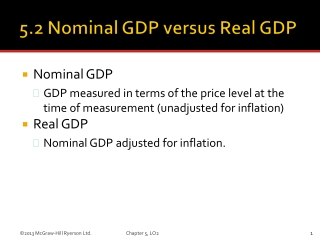DownloadDownload PresentationNominal GDP

# Nominal GDP

Télécharger la présentation## Nominal GDP

- - - - - - - - - - - - - - - - - - - - - - - - - - - E N D - - - - - - - - - - - - - - - - - - - - - - - - - - -
##### Presentation Transcript

1. Nominal GDP • GDP measured in terms of the price level at the time of measurement (unadjusted for inflation) • Real GDP • Nominal GDP adjusted for inflation. Chapter 5, LO2

2. Chapter 5, LO2

3. A measure of the price of a specified collection of goods and services, called a “market basket,” in a specific year as compared to the price of an identical (or highly similar) collection of goods and services in a reference year Chapter 5, LO2

4. Price index in specific year = price of market basket in specific year x 100 price of same market basket in base year • For example, if in year 2, price of basket is \$20 Price of same basket in base year is \$10, then price index, year 2 = (\$20/\$10) x 100 = 200. Chapter 5, LO2

5. For example, if in year 2, nominal GDP is \$140 and price index is 200, then Real GDP = (\$140/200) x 100 = \$70. Chapter 5, LO2

6. Chapter 5, LO2

7. For example, if in year 2, nominal GDP is \$140 and real GDP is \$70, then GDP Deflator = (\$140/\$70) x 100 = 200. Chapter 5, LO2

8. Table 5-6 Steps for Deriving Real GDP from Nominal GDP • Method 1: 1. Find nominal GDP for each year. 2. Compute a price index. 3. Divide each year’s nominal GDP by that year’s price index, then multiply by 100 to determine real GDP. Chapter 5, LO2

9. Table 5-6 Steps for Deriving Real GDP from Nominal GDP • Method 2: 1. Break down nominal GDP into physical quantities of output and prices for each year. 2. Find real GDP for each year by determining the dollar amount that each year’s physical output would have sold for if base-year prices had prevailed. Chapter 5, LO2

10. Links each year to the previous year through the use of both the prior-year prices and current-year prices. • For example, the calculation of the chain-weighted index would use both 2011 and 2012 prices to calculate real GDP growth in 2012. Since the 2011 chain-weighted index was arrived at using both 2010 and 2011 prices, the year 2010 is linked back - as the links of a chain are - to 2009, 2008 and previous years as well. Chapter 5, LO2

11. Chapter 5, LO2